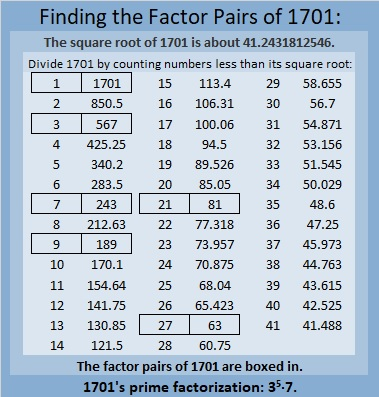# 1701 Is a Decagonal Number

Contents

### Today’s Puzzle:

There is a pattern to the decagonal numbers. Can you figure out what it is?### Factors of 1701:

1701 is divisible by nine because 1 + 7 + 0 + 1 = 9.

• 1701 is a composite number.
• Prime factorization: 1701 = 3 × 3 × 3 × 3 × 3 × 7, which can be written 1701 = 3⁵ × 7.
• 1701 has at least one exponent greater than 1 in its prime factorization so √1701 can be simplified. Taking the factor pair from the factor pair table below with the largest square number factor, we get √1701 = (√81)(√21) = 9√21.
• The exponents in the prime factorization are 5 and 1. Adding one to each exponent and multiplying we get (5 + 1)(1 + 1) = 6 × 2 = 12. Therefore 1701 has exactly 12 factors.
• The factors of 1701 are outlined with their factor pair partners in the graphic below.### More About the Number 1701:

1701 is the difference of two squares in SIX different ways.
851² – 850² = 1701,
285² – 282² = 1701,
125² – 118² = 1701,
99² – 90² = 1701,
51² – 30² = 1701, and
45² – 18² = 1701.

1701 is the 21st decagonal number because
21(4·21 – 3) =
21(84-3) =
21(81) = 1701.

There is decagonal number generating function:
x(7x+1)/(1-x)³ = x + 10x² + 27x³ + 52x⁴ + 85x⁵ + . . .

The 21st term of that function is 1701 x²¹.

This site uses Akismet to reduce spam. Learn how your comment data is processed.